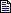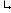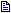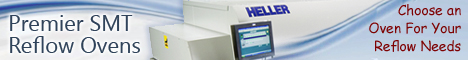Printed Circuit Board Assembly & PCB Design Forum

SMT electronics assembly manufacturing forum.

# Calculating Reflow SlopeWe currently use a KIC 2000 system to profile on our oven an... - Oct 05, 2004Are you following solder paste manufacturer's spec?? I know ... - Oct 05, 2004We are using the paste vendor's recommendations for TAL, pre... - Oct 05, 2004We do not know how your KIC2000 calculates slope. Here's ... - Oct 05, 2004The sampling rate on the KIC software can be changed by the ... - Oct 06, 2004

Calculating Reflow Slope | 5 October, 2004

We currently use a KIC 2000 system to profile on our oven and create our recipes. I had a customer in today reviewing our profiles, and he had concerns that we were exceeding 4 deg. c/sec. on our profiles. The process window parameters that we use by default were set to calculate the slope over 60 seconds, which resulted in a "good" slope of 1.1. However, if I change the time to calculate the slope over to 1 second (an extreme, I know), I get slopes of 4 and 5 which violate the 4 deg. C/sec. rule. What is the "standard" time to use to calculate a slope? Thanks.

Calculating Reflow Slope | 5 October, 2004

Are you following solder paste manufacturer's spec?? I know still some time it is hard to maintain slope particularly with less zone reflow oven.

Calculating Reflow Slope | 5 October, 2004

We are using the paste vendor's recommendations for TAL, preheat, etc. They do not give any indication on their datasheet for the time to use to calculate slope (calculate over 20 seconds, 60 seconds, etc. - not included in their data).

Calculating Reflow Slope | 5 October, 2004

We do not know how your KIC2000 calculates slope.

Here's an approach to calculating slope: * Subtract the lower temperature from the upper temperature. [Temp|upper-Temp|lower] = Temp|change * Subtract the time when the lower temperature was measured from the time when the upper temperature was measured. [Time|upper-Time|lower] = Time|change * Divide Temp|change by Time|change to determine slope.

Generally, people calculate slope over the entire time that the temperature is changing value, because doing that averages all the little variations in slope over the course of the measurement. Actually, you can use any time period to calculate the slope, because the slope of a straight line is constant along the entire line. You just need to use temperatures and elapsed times that correspond to one another.

See when you "change the time to calculate the slope over to 1 second", you need to reduce the temperature change calculation to correspond to the reduction in time. Otherwise, your slope seems to increase.

Calculating Reflow Slope | 6 October, 2004

The sampling rate on the KIC software can be changed by the user. The default setting is a sample rate of 60 seconds, so that, every 60 seconds the "old" measurement is subtracted from the "new" measurement to calculate the slope (i.e., start temp is 30C, end is 90C, over 60 seconds gives a slope of 1). At least, that is how it looks from the software settings. I know that there has to be some time range that is "best" in order to compensate for slight variations in the heat transfer characteristics of the oven and its interraction to the substrate and components, but not so long a time between samples that it masks a potential dangerous spike that could damage heat-sensitive devices. Has anyone else run into this sort of problem? What sampling rate can you recommend?